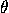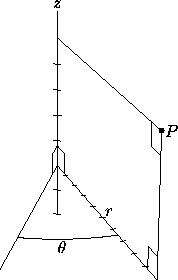# 9.2 Cylindrical Coordinates in Space

To define cylindrical coordinates, we take an axis (usually called the z-axis) and a perpendicular plane, on which we choose a ray (the initial ray) originating at the intersection of the plane and the axis (the origin). The coordinates of a point P are: the polar coordinates (r,) of the projection of P on the plane, and the coordinate z of the projection of P on the axis (Figure 1). See Section 1.3 for remarks on the values of r and.Figure 1: Among the possible sets (r,,z) of cylindrical coordinates for P are (10,30°,5) and (10,390°,5).

Next: 9.3 Spherical Coordinates in Space
Up: 9 Coordinate Systems in Space
Previous: 9.1 Cartesian Coordinates in SpaceThe Geometry Center Home Page

Silvio Levy
Wed Oct 4 16:41:25 PDT 1995

This document is excerpted from the 30th Edition of the CRC Standard Mathematical Tables and Formulas (CRC Press). Unauthorized duplication is forbidden.